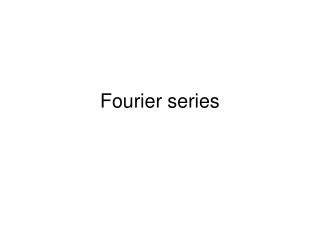DownloadDownload PresentationFourier series

# Fourier series

Télécharger la présentation## Fourier series

- - - - - - - - - - - - - - - - - - - - - - - - - - - E N D - - - - - - - - - - - - - - - - - - - - - - - - - - -
##### Presentation Transcript

1. Fourier series

2. The frequency domain • It is sometimes preferable to work in the frequency domain rather than time • Some mathematical operations are easier in the frequency domain • the human ear works on frequencies • Working in this domain really means the x axis is f and not t. • We need a method to convert time domain functions into frequency domain functions

3. Fourier Series • A sinusoid can be represented by • A sinusoid can be expressed as a sum of a sine and cosine at the same frequency but possibly different magnitudes independently of phase

4. Adding sinusoids with freq F results in a sinusoid with frequency F x(t)=sin(4πt) + 0.6cos(4πt)

5. x(t)=sin(4πt) + 0.6cos(4πt)

6. Any periodic waveform can be represented as an infinite sum of sine and cosine waves regardless of phase (as shown in the previous slide). This is the Fourier Series f(t) • Or more succinctly written as: • a0=const, an and bn are the amplitudes of the individual harmonics making up the periodic waveform

7. Discrete Fourier Transform DFT • The data we will use is sampled and an infinite number of samples is impractical • Works with non continuous non periodic functions • N time domain samples transform to N complex DFT values in the frequency domain

8. Periodic function generation • Remember that you created a sine wave digitally using:

9. The DFT is: • Where F is effectively a row matrix of size N • h is the harmonic • n is the time domain sample number • x(n) is the magnitude of the nth sample • N is the total number of samples

10. As each Fourier coefficient F(h) is complex so its magnitude and phase (with respect to the fundamental) need to be calculated:

11. Example • Consider 4 samples of a waveform from the time domain (from an a to d converter) {1,0,0,1}

12. Show that x(2)=0 and x(3)=1-j • So the DFT of a time vector {1,0,0,1}is a vector {2,1+j,0,1-j} • These coefficients are frequency independent as no account for frequency has been taken. We know that the second coefficient F(1) will represent the fundamental and the next one F(2) will be the next harmonic etc. If we need to find the fundamental frequency, we need to specify the coefficient in terms of sample frequency Fs where

13. Power phase diagram • Often wish to represent Fourier spectrum diagrammatically • Power is magnitude squared • Phase is angle • Line up power graph with phase graph • Plot actual frequencies is sample rate known • Usually only plot samples from 0 to N/2

14. From example x = {1,0,0,1}giving Fourier coefficients of {2,1+j,0,1-j} • If Fs=1000Hz, F1=1000/N=250, F2=500, F3=750 • Polar form of power and phase:

15. M 4 2 F 0 250 1k 500 750 90 45 0 -45 -90